# Speech recognition technology analysis: the voice becomes text is not so mysterious

Briefly explain to you how the voice changes into text. I hope this introduction will be understood by all students.

First, we know that sound is actually a wave. Common mp3, wmv and other formats are all compressed formats, which must be converted to uncompressed pure waveform files for processing, such as Windows PCM files, also known as wav files. In addition to a file header stored in the wav file, it is a point of the sound waveform. The figure below is an example of a waveform.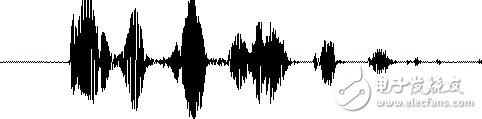Before starting speech recognition, it is sometimes necessary to cut off the silence at the head and end to reduce the interference to the subsequent steps. This silent cut operation is generally called VAD and requires some signal processing techniques.

To analyze the sound, the sound needs to be framed, that is, the sound is cut into small segments, and each small segment is called a frame. Framing operations are generally not simple cuts, but are implemented using moving window functions, which are not detailed here. There is generally overlap between frames, as shown below: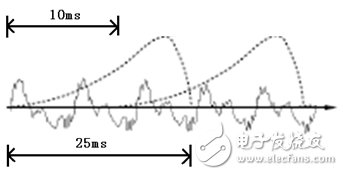In the figure, the length of each frame is 25 ms, and there is an overlap of 25-10 = 15 ms between every two frames. We call it frame division with frame length 25 ms and frame shift 10 ms. In the figure, the length of each frame is 25 ms, and there is an overlap of 25-10 = 15 ms between every two frames. We call it frame division with frame length 25 ms and frame shift 10 ms.

After framing, the voice becomes many small segments. But the waveform has almost no description ability in the time domain, so the waveform must be transformed. A common transformation method is to extract the MFCC features. According to the physiological characteristics of the human ear, each frame of the waveform is transformed into a multi-dimensional vector. It can be simply understood that this vector contains the content information of this frame of speech. This process is called acoustic feature extraction. In practical applications, there are many details in this step, and the acoustic characteristics are not limited to the MFCC, which is not described here.

So far, the sound has become a matrix of 12 rows (assuming 12-dimensional acoustic features), N columns, called the observation sequence, where N is the total number of frames. The observation sequence is shown in the following figure. In the figure, each frame is represented by a 12-dimensional vector, and the color depth of the color block indicates the size of the vector value.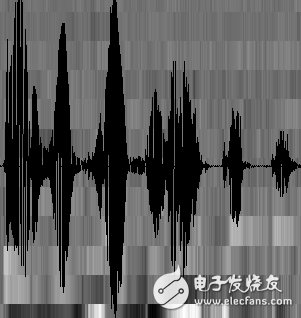Next, we will introduce how to turn this matrix into text. First, two concepts are introduced:

1. Phonemes: The pronunciation of words consists of phonemes. For English, a commonly used phoneme set is a set of 39 phonemes at Carnegie Mellon University, see The CMU Pronouncing DicTIonary. Chinese generally uses all initials and finals directly as phoneme sets. In addition, Chinese recognition is divided into tones and non-tones, which will not be described in detail.

2. Status: Here it is understood to be a more detailed phonetic unit than a phoneme. Usually a phoneme is divided into 3 states.

How does speech recognition work? Actually it is not mysterious at all, it is nothing more than:

The first step is to identify the frame as a state (difficulty);

The second step is to combine the states into phonemes;

The third step is to combine phonemes into words.

As shown below: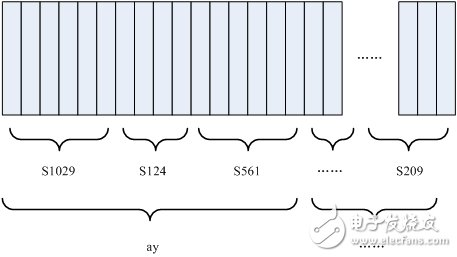In the figure, each small vertical bar represents a frame, several frames of speech correspond to a state, every three states are combined into a phoneme, and several phonemes are combined into a word. In other words, as long as you know which state each frame of speech corresponds to, the result of speech recognition will come out. In the figure, each small vertical bar represents a frame, several frames of speech correspond to a state, every three states are combined into a phoneme, and several phonemes are combined into a word. In other words, as long as you know which state each frame of speech corresponds to, the result of speech recognition will come out.

Which state does each frame phoneme correspond to? There is an easy way to think about which state corresponds to which state a frame has the highest probability. For example, in the following diagram, this frame has the highest probability of corresponding to the S3 state, so let this frame belong to the S3 state.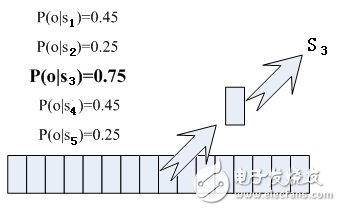Where do you read the probabilities used? There is something called an "acoustic model", which contains a large number of parameters. Through these parameters, you can know the probability of the frame and the state. The method of obtaining this large number of parameters is called "training", which requires the use of a huge amount of voice data. The method of training is cumbersome, and I will not talk about it here.

But there is a problem with this: every frame will get a status number, and finally the whole voice will get a bunch of messy status numbers. The status numbers between two adjacent frames are basically different. Suppose there are 1000 frames of speech, each frame corresponds to 1 state, and every 3 states are combined into one phoneme, then it will probably be combined into 300 phonemes, but this speech actually does not have so many phonemes. If you do so, the obtained status numbers may not be combined into phonemes at all. In fact, it should be reasonable that the states of adjacent frames are mostly the same because each frame is very short.

The common method to solve this problem is to use Hidden Markov Model (HMM). This thing sounds very deep, but it is actually very simple to use:

The first step is to build a state network.

The second step is to find the path that best matches the sound from the state network.

In this way, the results are limited to the pre-set network, which avoids the problem just mentioned, and of course brings a limitation. For example, the network you set includes only "Today is sunny" and "It is raining". The state path of the sentence, no matter what is said, the result of the recognition must be one of the two sentences.

What if you want to recognize arbitrary text? Make the network large enough to include any text path. But the larger the network, the harder it is to achieve better recognition accuracy. Therefore, according to the needs of the actual task, the size and structure of the network should be reasonably selected.

To build a state network is to expand from a word-level network to a phoneme network, and then to a state network. The process of speech recognition is actually to search for an optimal path in the state network. The probability that the voice corresponds to this path is the largest. This is called "decoding". The path search algorithm is a dynamic programming pruning algorithm, called Viterbi algorithm, used to find the global optimal path.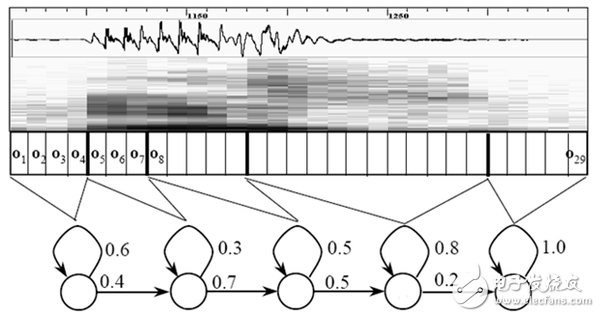The cumulative probability mentioned here consists of three parts, namely:

Observation probability: the probability of each frame and each state

Probability of transition: the probability that each state transitions to itself or to the next state

Language Probability: Probability based on language statistics

Among them, the first two probabilities are obtained from the acoustic model, and the last one is obtained from the language model. Language models are trained using a large amount of text, and the statistical rules of a language can be used to help improve recognition accuracy. The language model is very important. If the language model is not used, when the state network is large, the recognition result is basically a mess.

This basically completes the speech recognition process.

The above introduction is the traditional speech recognition based on HMM. In fact, the connotation of HMM is by no means as simple as the above is nothing more than a state network. The above text is just to make it easy for everyone to understand, not to pursue rigor.